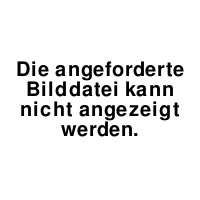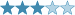## Cyclometer 234

(Eingestellt am 29. Oktober 2021, 04:02 Uhr von SirSchmoopy)

This is another entry in my series of puzzles using this concept of cycles as a constraint. I've gotten some feedback that these puzzles still take a while to understand, so I'm adding what I hope is a very thorough 6x6 example with some examples of the logic. I've also gone back an updated one of my earlier puzzles to include a walkthrough guide with some more illustrated examples.Definitions:

Let a cycle be the horizontal path of cells along a single row in the grid created by starting with a cell in column X with a digit is A, then going to column A with a digit of B, then going to column B whose digit is C, etc.

Let the order of a cycle be the number of unique digits contained in cells along a cycle.

For a concrete example: if a cell in column 1 contained an 8, then the next cell in the cycle would be the cell in column 8 for that row. Then, if that cell in column 8 had a 2 as its digit, the next cell in the cycle would be the cell in column 2 for that row, if column 2's cell contained a 1, then this would complete an order 3 cycle (8 -> 2 -> 1) .

Rules:

• Normal Sudoku rules apply
• Normal thermo rules apply
• Cyclometers: along with normal thermo rules, the orders of cycles along thermometers strictly increases starting from the bulb

6x6 Example:

Here is a 6x6 puzzle with a logical solution if you'd like to try something simpler. You can actually start this 6x6 by placing the entirety of row 4 and 5 without considering any other clues or even the given digits. I've given a walkthrough of this logic below highlighting the constraints of this ruleset wherever possible/trying to avoid using regular Sudoku.6x6 walkthrough:

Look at the 4 cell thermo beginning in R5C1

1. Three of these thermo cells are in the same row. Cycles cannot partially overlap (could two different cycles contain the same cell unless they were exactly the same? No/cycles are either completely identical or completely distinct on a given row. This is critical to solving the actual puzzle Try to prove that yourself if it doesn't seem obvious)

2. At the same time, there are only 6 cells in the row, so for there to be 3 distinct orders (orders strictly increase so they can't be the same along the thermo), you'd have to have exactly 1 order 1, 1 order 2, and one order 3 cycle in the row.

3. The order 1 cycle must start on the bulb. This makes R5C1 have an order of 1 -> its cell's digit is its column number -> it is a 1

4. As the order of R5C2 is a 2, its cell corresponds to the column number where the 2 is in R5. This is limited to columns 4,5,6, yet only a 4 is possible given normal thermo rules. (another key observation - R5C2 could never be a 3, as that would combine it with the cycle further up the thermo which must have a strictly greater order. This type of deduction is extremely important for the actual puzzle)

5. This then forces a 4 in R5C2 2 in R5C4, and a 5 and 6 along the rest of the thermo

6. R5C3 is order 3, so R5C5 cannot be a 3 (if it was, then it would form an order 2 cycle), so this finishes the row with a 6 in R5C5 and a 3 in R5C6

Next consider row 4

1. The bulb in R4C1 is not a 1, and therefore is not an order 1 cell. So it has an order of at least 2.

2. The 6 in R4C3 has an order of at least 4 (it's order is greater than R5C3 which is order 3)

3. As the total order of distinct cycles in a row is 6, this forces the bulb to be order 2 and 6 to be order 4 (This also means the tip of the thermo in R4C2 is part of the same cycle as R4C3). At this point, you know that in row 4, everything is in an order 2 or an order 4 cycle. Notably there are no order 1 cycles. This means that in row 4, a cell can never have a value equal to that cell's column number. This type of deduction is extremely important for the actual puzzle

4. As R4C1 is order 2, its cell's digit is the column where 1 is in row 4. This is in column, 4,5, or 6, but by normal thermo, this cell can be at most 4. This forces a 4 and 5 in the thermo.

5. The 4 in R4C1 is order 2, so R4C4 is a 1

6. R4C6 cannot be a 3, as it would form an order 2 cycle with R4C3. This finishes the rest of the row

Here is the finished 6x6. I've highlighted each of the cycles as a distinct color/shape in the row (cycles remain in a row, so 2 different rows having the same color/shape in this example isn't significant).Lösungscode: Row 6 + Column 6 (18 digits, no spaces)

Zuletzt geändert am 29. Oktober 2021, 04:38 Uhr

Gelöst von kaitoJD, Arashdeep Singh, RJBlarmo, Droi, Piatato, bolado, arskiy
Komplette Liste

### Kommentare

am 7. November 2021, 15:57 Uhr von Piatato
Great puzzle!

am 1. November 2021, 17:03 Uhr von Droi
@SirSchmoopy

I gave it a bit more thought, and I think "Loops" and "Length" are catchy, straightforward terms for this puzzle type, with a concept that is already familiar to anyone who has done programming at least haha. Just a suggestion, have a good one!

Zuletzt geändert am 1. November 2021, 16:47 Uhr

am 1. November 2021, 08:50 Uhr von Droi
Absolutely stunning! I love how mind bending the cycles are.

I will say it is a bit difficult without a designated solver since there are multiple layers in the puzzle, If anyone wants a tip, I'd suggest to use colors to keep track of what is the order of the cell.

I would love to see more of these, probably with a slightly different marker than a normal thermometer.

Also, the rules are written in a very mathematical way which would be a turn off for many people.

Maybe something like this?
"This puzzle contains *Cycles*. Each digit is a reference to another cell in the row, and each cycle is a set of digits that creates a loop in that row.

The Order of the Cycle is the number of digits it contains. (1-9)"

Good job and thanks for the puzzle!

[SirSchmoopy] - Thank you so much for the feedback! I love your suggestion for the ruleset description, and I think I will use that in the next puzzle that I create with these theme.

I do agree the tone is a bit mathematical, and while that was intentional since it was inspired by direct analogue here - the https://en.wikipedia.org/wiki/Cyclic_permutation I realize now that might make it seem less approachable than it actually is.

am 31. Oktober 2021, 23:56 Uhr von uvo_mod
Labels angepasst.

am 29. Oktober 2021, 17:29 Uhr von Arashdeep Singh
Another beautiful puzzle. Loved it. :)

am 29. Oktober 2021, 06:02 Uhr von kaitoJD
Excellent! I believe the more in-depth explanation is very good. I think its really nice that you highlight where the crucial deductions are. It also provides a nice segue into the actual puzzle.

 Schwierigkeit:Bewertung: N/A Gelöst: 7 mal Beobachtet: 1 mal ID: 00083F

Lösungscode:

## Anmelden